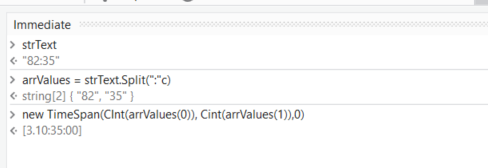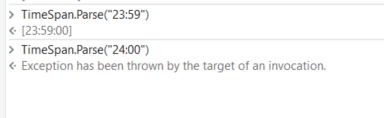# B>=A Compare column value

Testing_forum.xlsx (8.8 KB)
Hi
I have excel and i want B>=A then create excel and put the data in excel

What is 82:35
82:35

These are not valid times, what is the data type that you are referring to herein.

You can loop through the datatable.

Expecting that the values are in correct format you write something like

`TimeSpan.Parse(currentRow(1).ToString)>=TimeSpan.Parse(currentRow(0).ToString)`

This would work for you.
For Linq

filteredRecords= dtDetails.asEnumerable.Where(function (x) Timespan.Parse(x(1).ToString)>=Timespan.Parse(x(0).toString)).CopyToDataTable

Thanks

Happy Automation!

lets assume hours:minutes

we can parse it within a Timespan and then doing the comparemyTS1 = new TimeSpan(CInt(arrValues(0)), Cint(arrValues(1)),0)

Condition: myTS2 >= myTs1

We are forced to do this split as Timespan parse end on 23:59@ppr -This is minute and second

so we can do:
myTS1 = `new TimeSpan(0, CInt(arrValues(0)), Cint(arrValues(1)))`

Read Range into a datatable then use the Filter Datatable activity to keep the rows you want. Then Write Range back to a new Excel file.